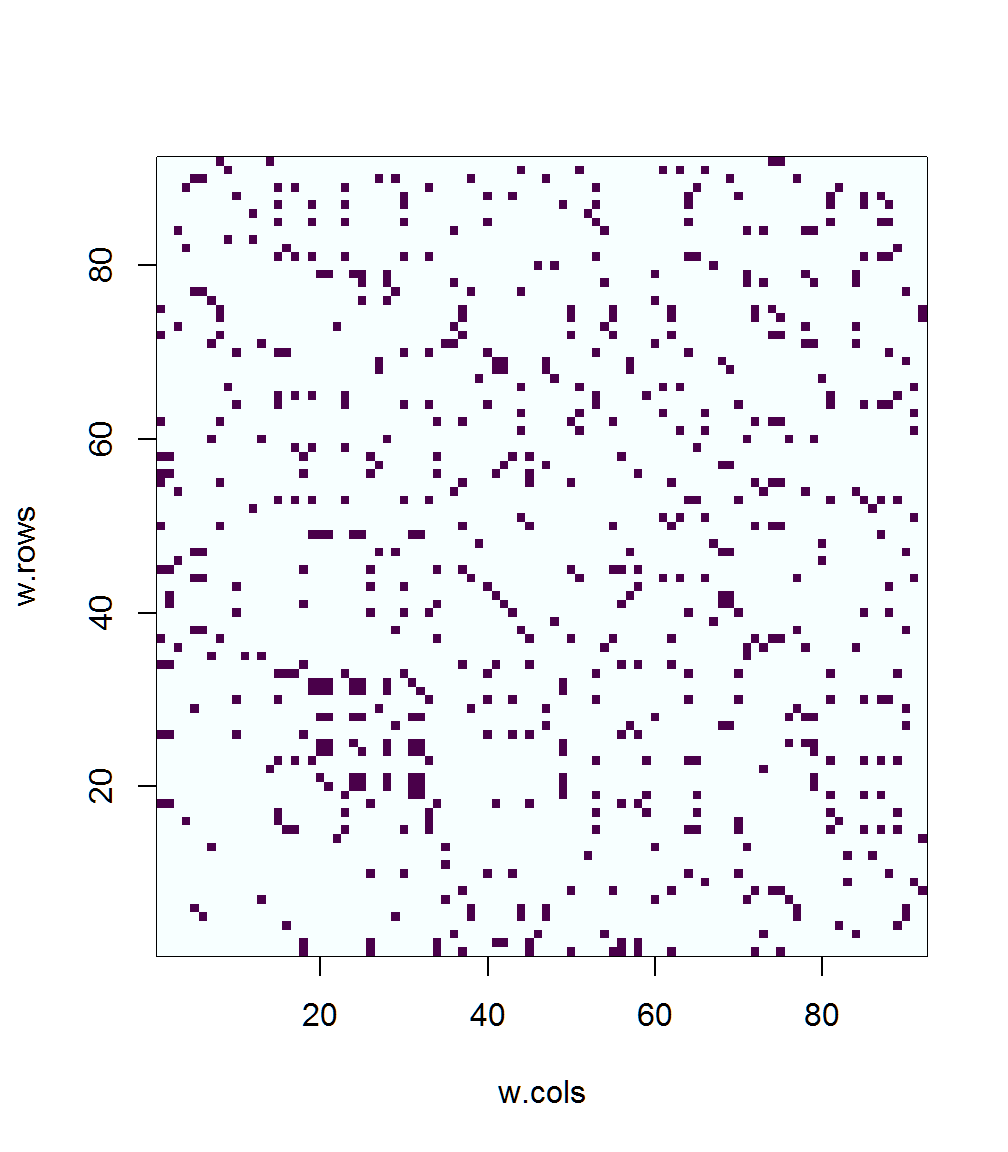# Spatial neighbors

Spatial neighbors are those points in a grid or irregular array that are “close” to one another, where “close” can mean adjacent or within some particular range of distances. Spatial neighbors can be obtained using the spdep package (by Roger Bivand and Luc Anselin). Some preliminaries:

library(maptools)
## Checking rgeos availability: TRUE
library(RColorBrewer)
library(spdep)
## Loading required package: Matrix
## read a county outline shape file
attach(orstationc)

# station location matrix
orstationc.loc <- cbind(orstationc$lon, orstationc$lat)

# plot the county outline shape file and the variable
plot(orotl.shp, xlim=c(-125, -115), ylim=c(42,47))

points(orstationc.loc, pch=16, cex=1.2)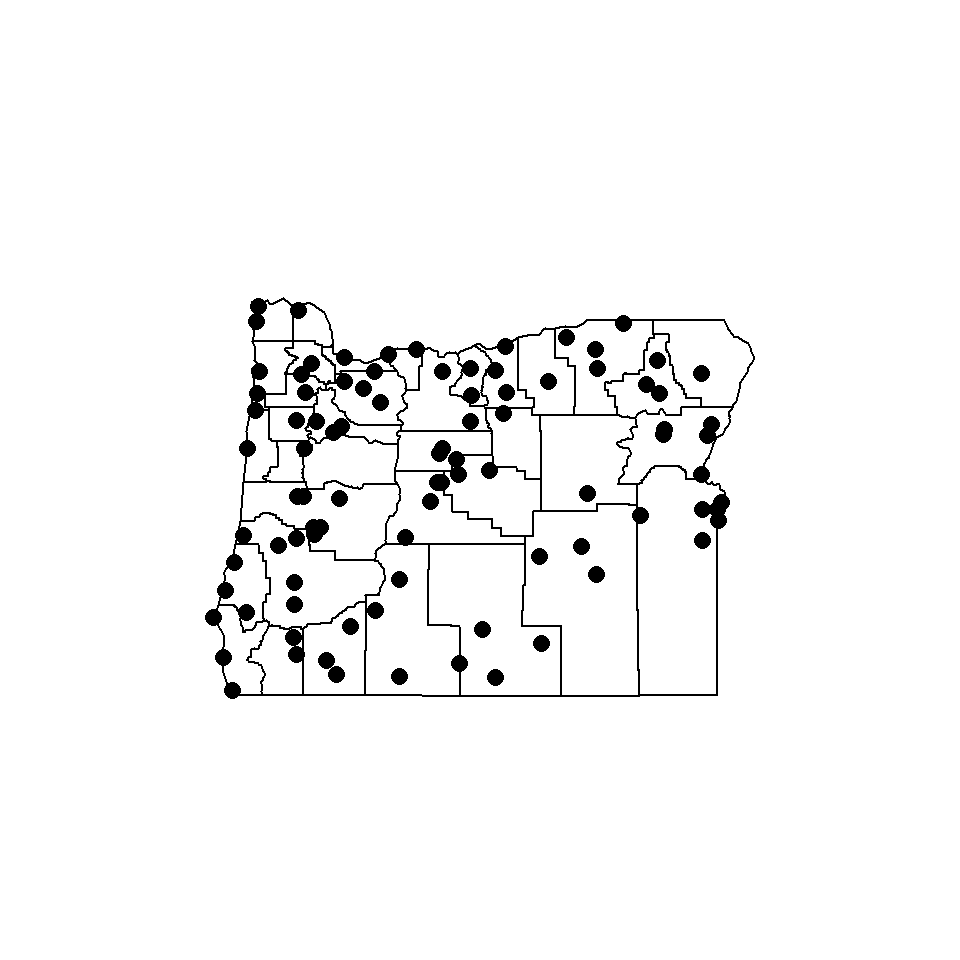#### Nearest-neighbor spatial neighbors, adjacency illustrated by line segments.

Adjacent points are sometimes referred to as “first-order lag” points, and are illustrated by line segments drawn between “neighboring” points.

# k nearest neighbors
k <- 4
nn <- knearneigh(orstationc.loc, k, longlat=T)
orstationc.neighbors.knn <- knn2nb(nn)
plot(orotl.shp, xlim=c(-125, -115), ylim=c(42,47))
plot(orstationc.neighbors.knn, orstationc.loc, add=T, lwd=2, col="magenta")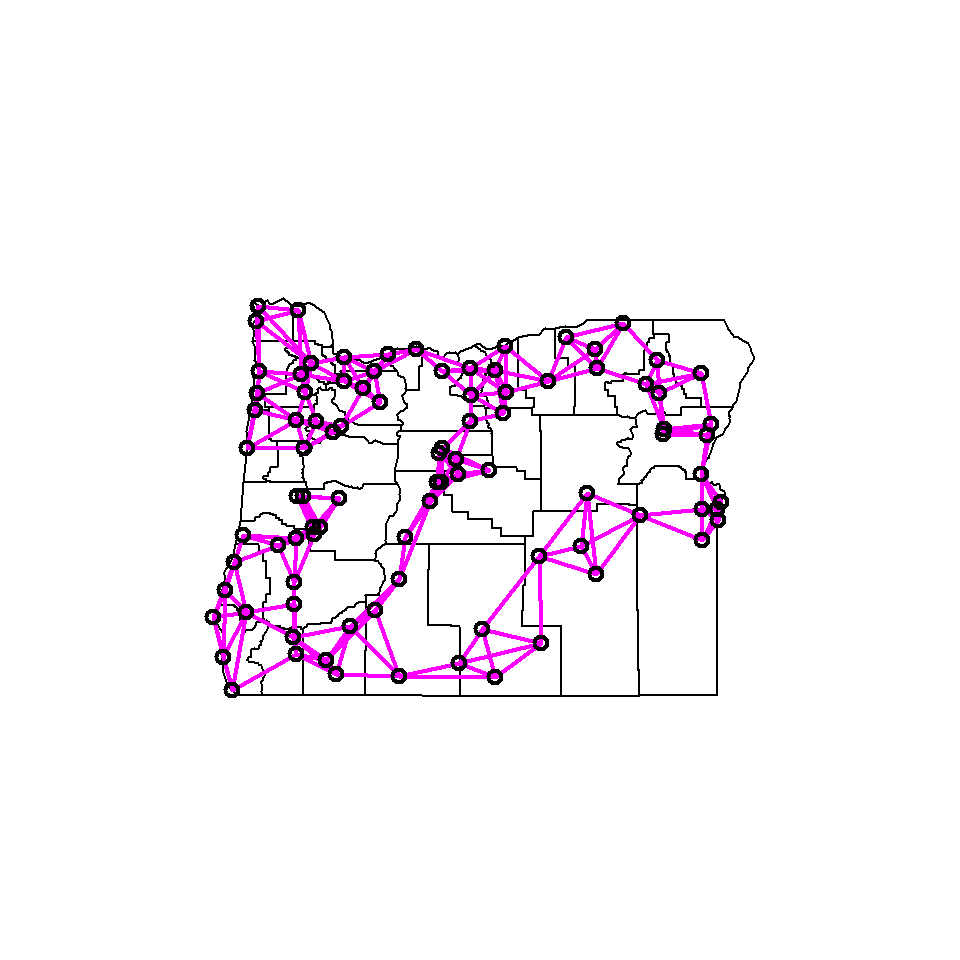#### Distance-based neighbors

# distance neighbors
d <- 80
orstationc.neighbors.dist <- dnearneigh(orstationc.loc, 0, d, longlat=TRUE)
plot(orotl.shp, xlim=c(-125, -115), ylim=c(42,47))
plot(orstationc.neighbors.dist, orstationc.loc, add=T, lwd=2, col="blue")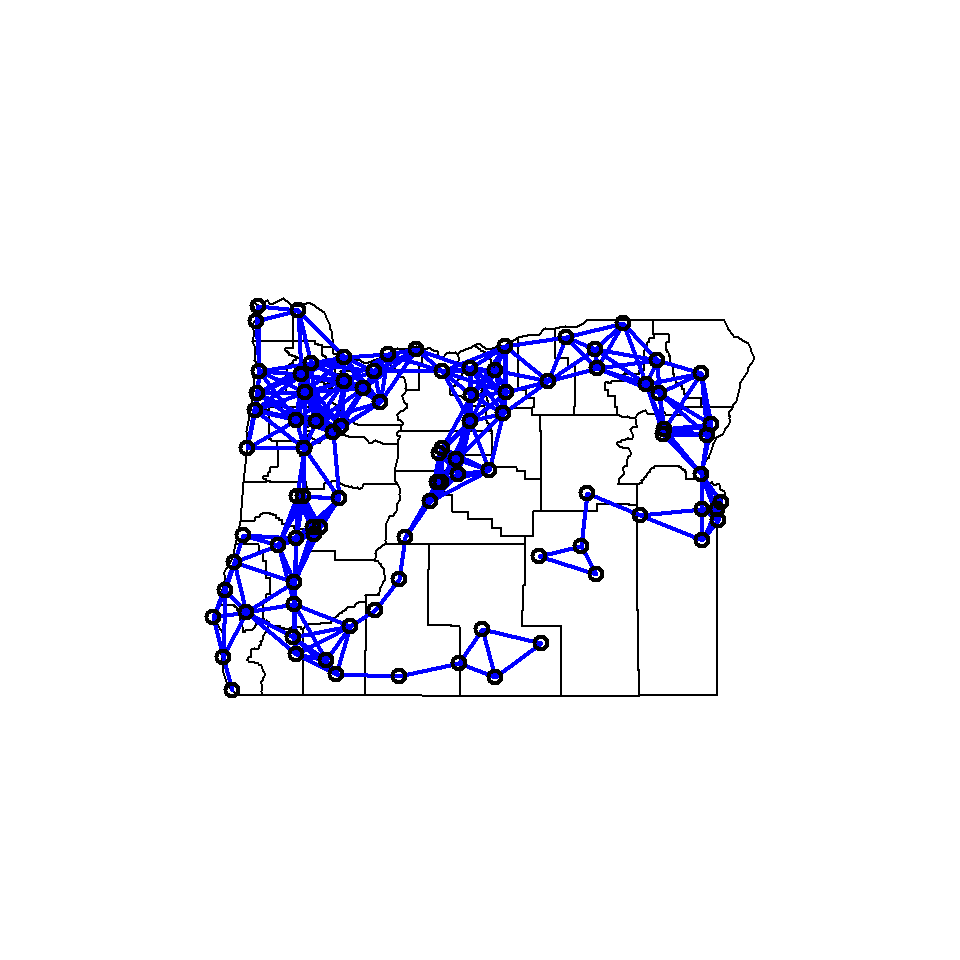The spatial-neighbor object can be summarized using the summary() function, which provides information on the nature of the links among points.

# summary description of a spatial-neighbor object
summary(orstationc.neighbors.dist, orstationc.loc)
## Neighbour list object:
## Number of regions: 92
## Number of nonzero links: 634
## Percentage nonzero weights: 7.490548
## Average number of links: 6.891304
##
##  1  2  3  4  5  6  7  8  9 10 11 12 13
##  1  7  7  5  9 12  5 20 11  7  1  5  2
## 1 least connected region:
## 2 most connected regions:
## 15 53 with 13 links
##    Min. 1st Qu.  Median    Mean 3rd Qu.    Max.
##  0.0525  0.4072  0.5730  0.5612  0.7219  1.0170
##
##   The decimal point is 1 digit(s) to the left of the |
##
##    0 | 557777778888
##    1 | 0011223333
##    1 | 777799999999
##    2 | 00001122223333334444
##    2 | 555566666677777788888888889999999999
##    3 | 00001111222222333333333333444444
##    3 | 5555667777777777777777777788889999
##    4 | 11112222222222223333334444444444
##    4 | 55555555556666666666667777777777888899999999
##    5 | 000000000000001111111122222222223333333333333344444444
##    5 | 555555555555555555666677777777777777778888999999999999
##    6 | 000000000000000000000022222222222233334444444444444444
##    6 | 5555555555555555556666667777777777888888889999
##    7 | 00000000000011111111222222222222222222333344444444
##    7 | 5555555555556677777777777788888888888888888899999999
##    8 | 00001111112222333333444444
##    8 | 55555555556666666677777777
##    9 | 00000011222222333333444444
##    9 | 556677777777
##   10 | 22

In addition looking at the first-order lags, it is also possible to look at second and higher-order lags:

# plotting second-order lags on top of the first-order lags
col.lags <- nblag(orstationc.neighbors.knn, 2)
plot(orotl.shp, xlim=c(-125, -115), ylim=c(42,47))
plot(col.lags[], orstationc.loc, add=T, lwd=2, col="green", lty=2)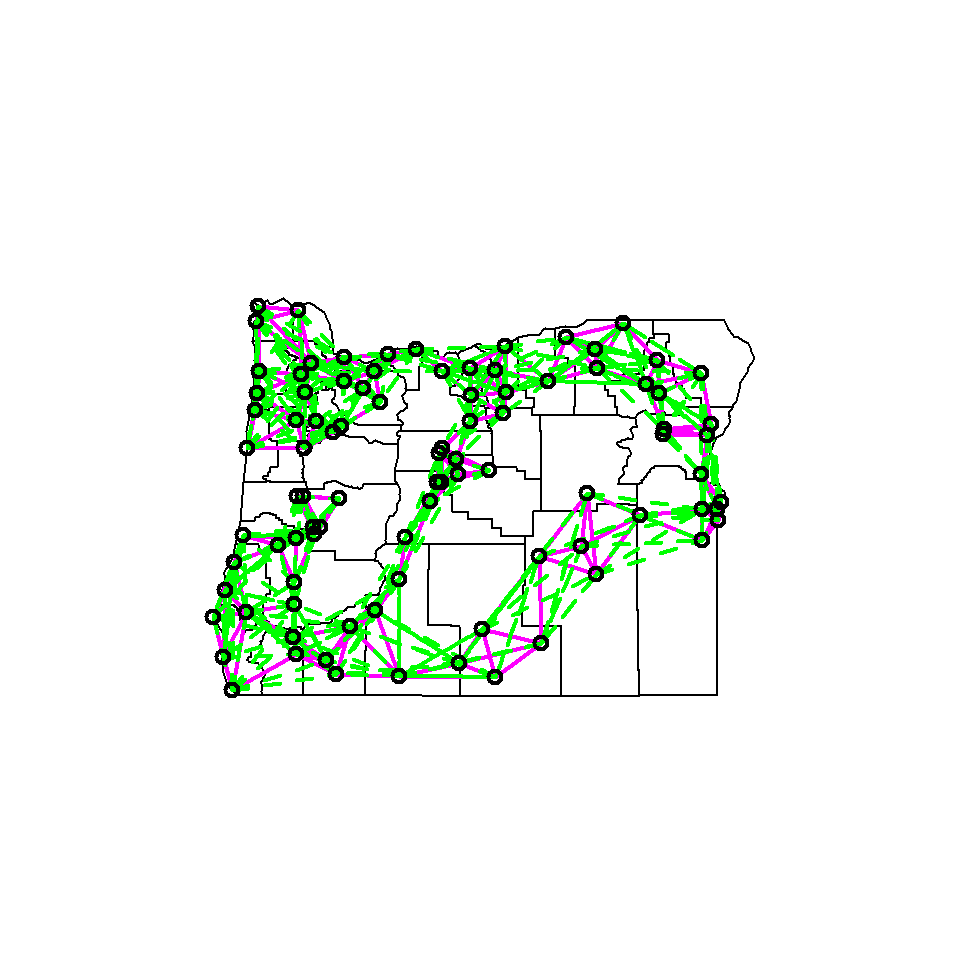The spatial-neighbors matrix can be visualized using the nb2mat() and image() functions:

Here’s what W looks like for nearest-neighbors (note that the matrix is not symmetrical).

# spatial weights matrix
w.cols <- 1:92
w.rows <- 1:92

w.mat.knn <- nb2mat(orstationc.neighbors.knn, zero.policy=TRUE)
image(w.cols, w.rows, w.mat.knn, col=brewer.pal(3,"BuPu"))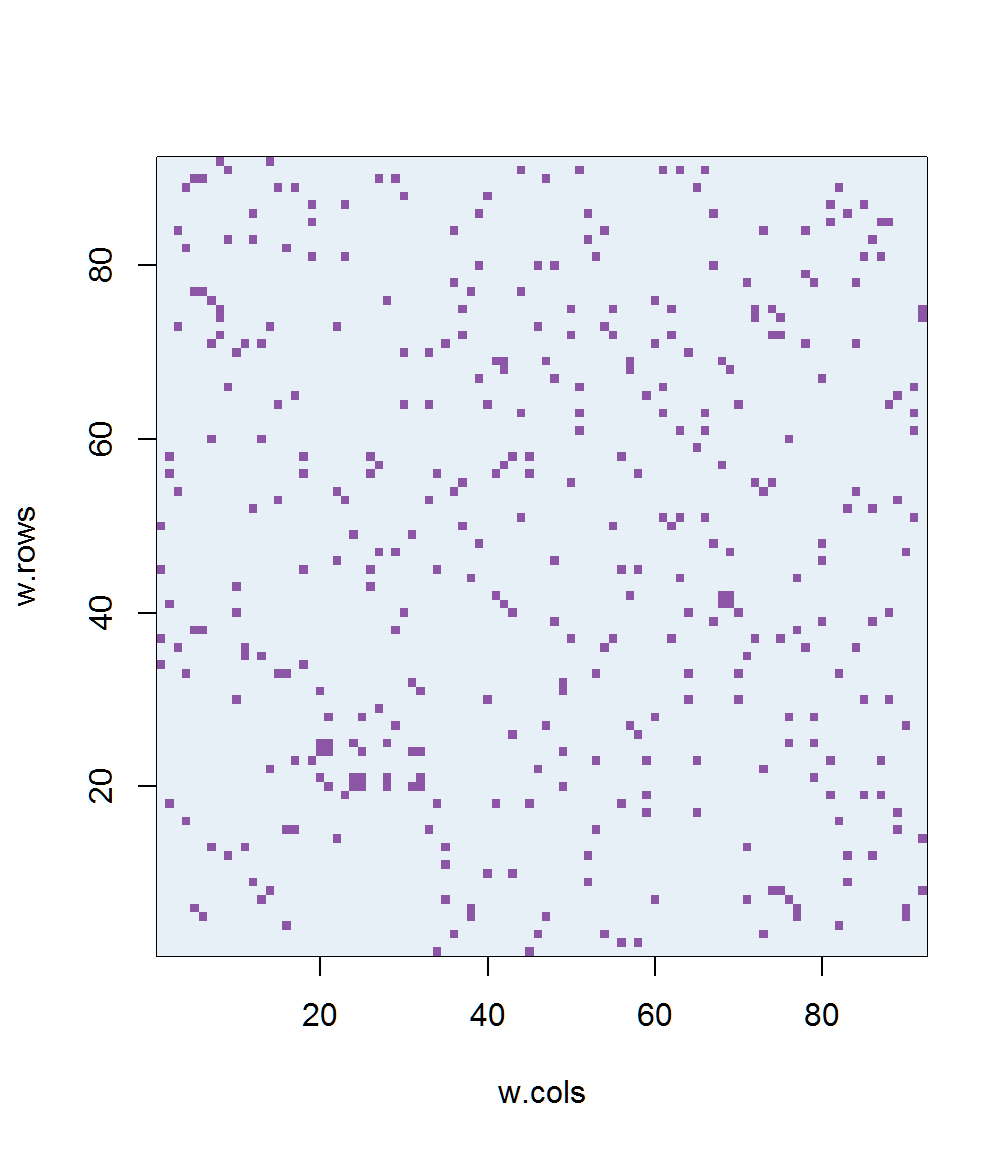Here’s W for distance-based neighbors. Again the matrix is not symmetrical, because it has been “row-standardized”.

w.mat.dist <- nb2mat(orstationc.neighbors.dist, zero.policy=TRUE)
image(w.cols, w.rows, w.mat.dist,col=brewer.pal(9,"BuPu"))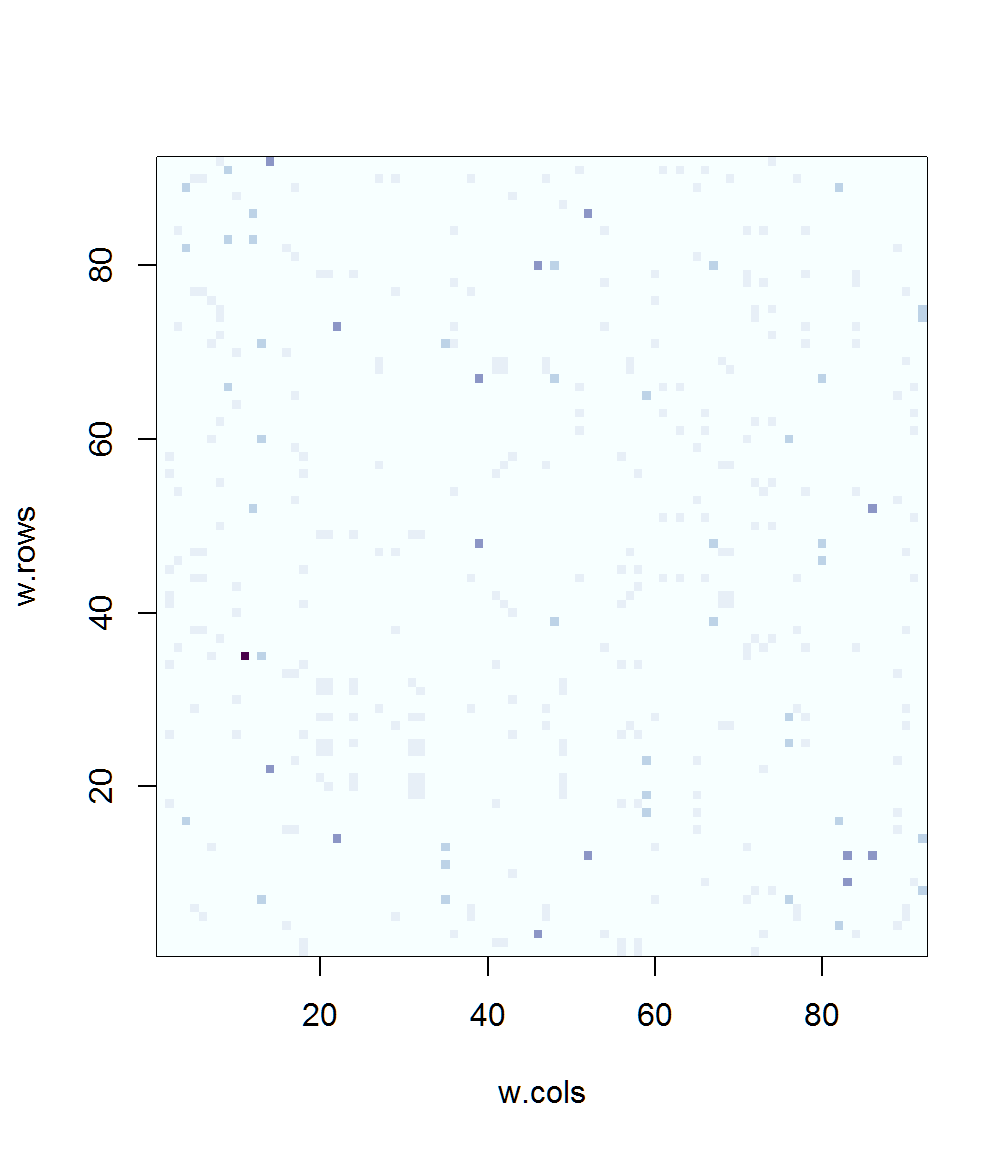The binary contiguity matrix can be visualzed by specifying style="B". Note that this matrix is symmetrical.

w.mat.dist <- nb2mat(orstationc.neighbors.dist, zero.policy=TRUE, style="B")
image(w.cols, w.rows, w.mat.dist,col=brewer.pal(9,"BuPu"))